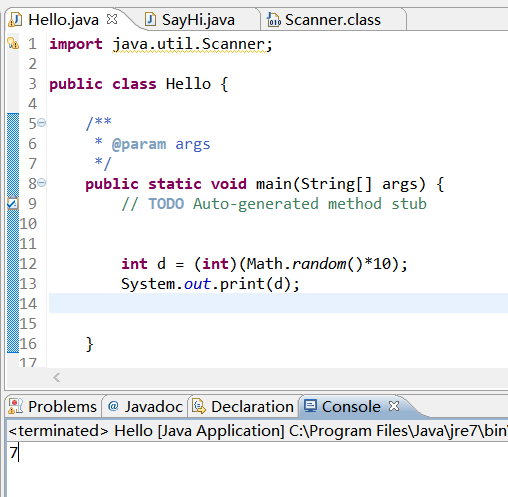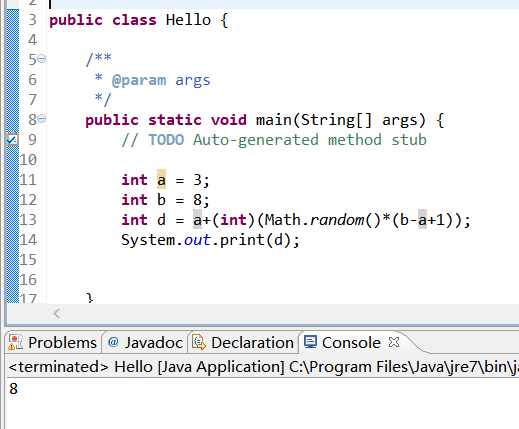• 生成0-9之间的随机数 用法 (int)(Math.random()*10) 例子 生成从0到n的随机整数 int num=(int)(Math.random()*(n+1); 生成从a到b的随机整数 说明 a是取值的下限范围 b是取值的上限范围 需求期望随机...
生成随机数
为什么需要随机数
有了随机数，程序会更加的生活
变化的数据，让程序有了活性
生成随机小数

用法


Math.random()



效果

生成0-1之间的随机小数，不包含1

例子生成0-9之间的随机数

用法


(int)(Math.random()*10)



例子生成从0到n的随机整数

int num=(int)(Math.random()*(n+1);


生成从a到b的随机整数

说明

a是取值的下限范围
b是取值的上限范围
需求期望随机值在a到b之间的整数
随机出来的数据是有可能得到a或者b的

用法


int num=a+(int)(Math.random()*(b-a+1));



例子展开全文• 按概率生成随机数Java版本
                思想什么的可以参看http://blog.csdn.net/slowgrace/archive/2009/03/25/4022632.aspx 如果要控制1个数落在某个区间的概率，比如要求在sngBegin和sngEnd之间生成一个随机数，这个随机数落在sngPB和sngPE之间的概率是P%。有两种方法，以第二种方法为好。先说第一种方法，要点是：（1）由于sngPB和sngPE将整个区间分成3部分，所以先分别计算随机数落在3部分的概率。落在sngPB和sngPE之间的概率是P%，这是已知的。余下的两个区间的总和概率是(1-p%),分到各个区间的概率按它们的长度分成。 （2）然后根据3个概率得到一个区间划分，落在第一个区间的，就在sngPB和sngPE之间生成一个随机数；落在第二个区间的，就是[sngBegin, sngPB]里生成随机数；落在第3个区间的，就在[sngPE,sngEnd]之间生数。    这个办法有个问题，就是用了两次随机数，这样实际上影响了它的随机性。Tiger_Zhao建议的第二种方法则没有这个问题，做法是：多个段有不同权重时其实可以映射成相同权重（缩放 [sngPB, sngPE] 区间，相对调整 sngEnd），这样只要一次 Rnd() 就可以完成，代码如下。   Random rand = new Random();  public static void main (String args[]) {    double bytP = 0.01;  double sngBegin = 1;  double sngEnd = 5;  double sngPB = 2;  double sngPE = 3;                Rand r = new Rand();                for (int i = 0; i < 1000; i++) {                  System.out.println(r.GetRndNumP(sngBegin, sngEnd, sngPB, sngPE, bytP));        } }     /**     * 按照一定概率进行随机<br>     * <br>     * @param pSngBegin 随机数范围的开始数字     * @param pSngEnd 随机数范围结束数字     * @param pSngPB 要随机的数字的开始数字     * @param pSngPE 要随机的数字的结束数字     * @param pBytP 要随机的数字随机概率     * @return 按照一定概率随机的数字     */ public double GetRndNumP(double pSngBegin,                     double pSngEnd,                     double pSngPB,                     double pSngPE,                     double pBytP) {    double sngPLen;    double sngTLen; //total length    double sngIncreased; //需要缩放的长度    double sngResult;    sngPLen = pSngPE - pSngPB;       sngTLen = pSngEnd - pSngBegin;          if ((sngPLen / sngTLen) * 100 == pBytP ) {            return GetRandomNum(pSngBegin, pSngEnd);           } else {            // ((sngPLen + sngIncreased) / (sngTLen + sngIncreased)) * 100 = bytP      sngIncreased = ((pBytP / 100) * sngTLen - sngPLen) / (1 - (pBytP / 100));            // 缩放回原来区间         sngResult = GetRandomNum(pSngBegin, pSngEnd + sngIncreased);            if (pSngBegin <= sngResult && sngResult <= pSngPB) {              return sngResult;             } else if (pSngPB <= sngResult && sngResult <= (pSngPE + sngIncreased)) {              return pSngPB + (sngResult - pSngPB) * sngPLen / (sngPLen + sngIncreased);             } else if ((pSngPE + sngIncreased) <= sngResult && sngResult <= (pSngEnd + sngIncreased )) {              return sngResult - sngIncreased;             }     }          return 0f; }  public double GetRandomNum(double pSngBegin, double pSngEnd) {    return (pSngEnd - pSngBegin) * Math.random() + pSngBegin; }            再分享一下我老师大神的人工智能教程吧。零基础！通俗易懂！风趣幽默！还带黄段子！希望你也加入到我们人工智能的队伍中来！https://blog.csdn.net/jiangjunshow


展开全文• java随机生成随机数 Generating a series of random numbers is one of those common tasks that crop up from time to time. In Java, it can be achieved simply by using the java.util.Random class. 生成一...
java随机生成随机数
Generating a series of random numbers is one of those common tasks that crop up from time to time. In Java, it can be achieved simply by using the java.util.Random class.

生成一系列随机数是不时出现的常见任务之一。 在Java中 ，只需使用java.util.Random类即可实现。

The first step, as with the use of any API class, is to put the import statement before the start of your program class:

与使用任何API 类一样 ，第一步是将import语句放在程序类开始之前：

Next, create a Random object:

接下来，创建一个Random对象：

The Random object provides you with a simple random number generator. The methods of the object give the ability to pick random numbers. For example, the nextInt() and nextLong() methods will return a number that is within the range of values (negative and positive) of the int and long data types respectively:

Random对象为您提供了一个简单的随机数生成器。 对象的方法可以选择随机数。 例如，nextInt()和nextLong()方法将分别返回int和long数据类型的值(负和正)范围内的数字：

The numbers returned will be randomly chosen int and long values:

返回的数字将是随机选择的int和long值：
从一定范围内挑选随机数 (  Picking Random Numbers From a Certain Range  )

Normally the random numbers to be generated need to be from a certain range (e.g., between 1 to 40 inclusively). For this purpose, the nextInt() method can also accept an int parameter. It denotes the upper limit for the range of numbers. However, the upper limit number is not included as one of the numbers that can be picked. That might sound confusing but the nextInt() method works from zero upwards. For example:

通常，要生成的随机数必须在某个范围内(例如，包括1到40之间)。 为此，nextInt()方法还可以接受一个int参数。 表示数字范围的上限。 但是，上限编号不作为可以选择的编号之一。 这听起来可能令人困惑，但是nextInt()方法从零开始工作。 例如：

will only pick a random number from 0 to 39 inclusively. To pick from a range that starts with 1, simply add 1 to the result of the nextInt() method. For example, to pick a number between 1 to 40 inclusively add one to the result:

只会选择一个从0到39(含)之间的随机数。 要从以1开头的范围中进行选择，只需将nextInt()方法的结果加1。 例如，要选择一个介于1到40之间(包括1和40)的数字，请在结果中加上一个：

If the range starts from a higher number than one you will need to:

如果范围从大于1的数字开始，则需要：
minus the starting number from the upper limit number and then add one. 从上限编号减去起始编号，然后再加一个。 add the starting number to the result of the nextInt() method. 将起始编号添加到nextInt()方法的结果中。

For example, to pick a number from 5 to 35 inclusively, the upper limit number will be 35-5+1=31 and 5 needs to be added to the result:

例如，要选择一个介于5到35之间的数字，上限数字将为35-5 + 1 = 31，并且需要在结果中加上5：
随机类别的随机性如何？ (  Just How Random Is the Random Class?  )

I should point out that the Random class generates random numbers in a deterministic way. The algorithm that produces the randomness is based on a number called a seed. If the seed number is known then it's possible to figure out the numbers that are going to be produced from the algorithm. To prove this I'll use the numbers from the date that Neil Armstrong first stepped on the Moon as my seed number (20th July 1969) :​

我应该指出，Random类以确定性方式生成随机数。 产生随机性的算法基于一个称为种子的数字。 如果知道种子编号，则可以找出将要从算法生成的编号。 为了证明这一点，我将使用尼尔·阿姆斯特朗第一次踏上月球的日期作为我的种子编号(1969年7月20日)：

No matter who runs this code the sequence of "random" numbers produced will be:

无论是谁运行此代码，产生的“随机”数字序列都将是：

By default the seed number that is used by:

默认情况下，以下对象使用的种子编号：

is the current time in milliseconds since January 1, 1970. Normally this will produce sufficiently random numbers for most purposes. However, note that two random number generators created within the same millisecond will generate the same random numbers.

是自1970年1月1日以来的当前时间(以毫秒为单位)。通常，对于大多数用途，这将产生足够的随机数。 但是，请注意，在同一毫秒内创建的两个随机数生成器将生成相同的随机数。

Also be careful when using the Random class for any application that must have a secure random number generator (e.g., a gambling program). It might be possible to guess the seed number based on the time the application is running. Generally, for applications where the random numbers are absolutely critical, it's best to find an alternative to the Random object. For most applications where there just needs to be a certain random element (e.g., dice for a board game) then it works fine.

将Random类用于必须具有安全随机数生成器的任何应用程序(例如，赌博程序)时，也要小心。 可能可以根据应用程序的运行时间猜测种子编号。 通常，对于随机数绝对重要的应用程序，最好找到随机对象的替代方法。 对于大多数只需要某个随机元素(例如，棋盘游戏的骰子)的应用程序来说，它可以正常工作。
翻译自: https://www.thoughtco.com/how-to-generate-random-numbers-2034206java随机生成随机数
展开全文算法 python 人工智能 机器学习
• Java 生成随机数 Java 中有两种生成随机数的方式： 利用 Math 类中 random() 方法 利用 Random 类。 Matn.random() 随机生成 0≤x&amp;lt;10 \leq x &amp;lt; 10≤x&lt;1 的小数。 ...
Java 生成随机数
随机数在实际中使用很广泛，比如要随即生成一个固定长度的字符串、数字。或者随即生成一个不定长度的数字、或者进行一个模拟的随机选择等等。Java提供了最基本的工具，可以实现生成伪随机数。
Java 中主要有两种生成随机数的方式：

利用 Math 类中的 random() 方法。
利用 Random 类。

Math.random()
随机生成 $0 \leq x < 1$ 的小数，即在 $[0,1)$ 之间取值。
应用
随机生成 $m \sim n$ 之间的整数，包括 m 和 n 。
公式： $(int)((Math.random()\ast(n-m+1))+1)$
/*
* 随机生成 m ~ n 之间的整数。
* m = 10, n = 20
* (int) (Math.random() * (20 - 10 + 1) + 10) = (int) (Math.random() * 11 + 10)
*/
int m = 10, n = 20, x = 0;
for (int i = m; i <= n; i++) {
do {
x = (int) (Math.random() * (n - m + 1) + m);
System.out.print(x + " ");
} while (x != i);
System.out.println();
}

【结果】
13 14 12 20 18 17 19 20 19 12 14 17 16 13 15 20 11 14 16 11 10
18 20 15 15 17 19 14 12 17 16 18 17 20 14 11
17 20 12
13
19 13 19 15 17 11 14
10 11 12 10 18 16 18 19 19 20 16 14 17 19 16 10 12 20 18 13 10 15
11 13 11 13 16
11 19 13 10 12 13 13 14 16 11 20 18 18 15 14 11 12 13 17
15 20 13 20 16 12 13 11 10 16 13 11 20 18
17 19
18 10 13 16 11 20

Math.random() 本身是用 Random 类实现的。
public static double random() {
return RandomNumberGeneratorHolder.randomNumberGenerator.nextDouble(); // 返回一个伪随机数，在 0.0 到 1.0 之间均匀分布的 double 值。
}
private static final class RandomNumberGeneratorHolder {
static final Random randomNumberGenerator = new Random();
}

Random 类
构造方法

默认构造方法：Random random = new Random()
指定种子数字：Random random = new Random(see)
等价于 Random random = new Random() + random.setSeed(seed)

在进行随机时，随机算法的起源数字称为种子数 (seed) ，在种子数的基础上进行一定的变换，从而产生需要的随机数字。
相同种子数的 Random 对象，相同次数生成的随机数字是完全相同的。也就是说，两个种子数相同的 Random 对象，第一次生成的随机数字完全相同，第二次生成的随机数字也完全相同。
Random 类两种构造方法的差别：

不带种子。此种方式将会返回随机的数字，每次运行结果不一样
带种子。此种方式，无论程序运行多少次，返回结果都是一样的

两种方式的差别

Random类的说明：此类的实例用于生成伪随机数流，此类使用 48 位的种子，该种子可以使用线性同余公式对其进行修改。如果用相同的种子创建两个 Random 实例，则对每个实例进行相同的方法调用序列，它们将生成并返回相同的数字序列。为了保证实现这种特性，我们为类 Random 指定了特定的算法。为了 Java 代码的完全可移植性，Java 实现必须让类 Random 使用此处所示的所有算法。但是允许 Random 类的子类使用其他算法，只要其符合所有方法的常规协定即可。
如果没有提供种子数，Random 实例的种子数将是当前时间的毫秒数，可以通过 System.currentTimeMillis() 来获得当前时间的毫秒数。

public Random() {
this(System.currentTimeMillis());
}
public Random(long seed);

Random 类中的常用方法
Random 类中的方法比较简单，每个方法的功能也很容易理解。需要说明的是，Random 类中各方法生成的随机数字都是均匀分布的，也就是说区间内部的数字生成的几率是均等的。

public void setSeed(long seed)
该方法的作用是重新设置 Random 对象中的种子数。设置完种子数以后的 Random 对象和相同种子数使用 new 关键字创建出的 Random 对象相同。

public boolean nextBoolean()
该方法的作用是生成一个随机的 boolean 值，生成 true 和 false 的值几率相等，各有 50% 的几率。

public int nextInt()
该方法的作用是生成一个随机的 int 值，该值介于 int 的区间，也就是 $-2^{31}\sim-2^{31}-1$ 之间。

public int nextInt(int n)
该方法的作用是生成一个随机的 int 值，该值介于 [0,n) 的区间，也就是 0 到 n 之间的随机 int 值，包含 0 而不包含 n 。

public int nextLong()
该方法的作用是生成一个随机的 long 值，该值介于 long 的区间，也就是 $-2^{63} \sim -2^{63}-1$ 之间。

public double nextFloat()
该方法的作用是生成一个随机的 Floa 值，数值介于 [ 0.0 , 1.0 ) 之间，也就是 0 到 1 之间的随机小数，包含 0.0 而不包含 1.0 。

public double nextDouble()
该方法的作用是生成一个随机的 double 值，数值介于 [ 0.0 , 1.0 ) 之间，也就是 0 到 1 之间的随机小数，包含 0.0 而不包含 1.0 。

public double nextGaussian()
该方法的作用是生成一个随机的 double 值，其规律服从平均值为 0.0 ，标准偏差为 1.0 的高斯 (“正”) 分布。

Random 类的部分函数：
public class Random {
public Random(); // 创建一个新的随机数生成器。
public Random(long seed); // 使用单个 long 种子创建一个新的随机数生成器。
synchronized public void setSeed(long seed); // 使用单个 long种子设置此随机数生成器的种子。
public void nextBytes(byte[] bytes) ; // 生成随机字节并将它们放入用户提供的字节数组中。
protected int next(int bits); // 生成下一个伪随机数。
public boolean nextBoolean(); // 从该随机数生成器的序列返回下一个伪随机数，均匀分布的 boolean 值。
public int nextInt(); // 从这个随机数生成器的序列返回下一个伪随机数，均匀分布的 int 值。
public int nextInt(int bound); // 从该随机数发生器的序列返回下一个伪随机数，均匀分布介于 0 (含) 和指定值 (不包括) 的 int 值。
public long nextLong(); // 从该随机数发生器的序列返回下一个伪随机数，均匀分布的 long 值。
public float nextFloat(); // 从该随机数生成器的序列返回下一个伪随机数，在 0.0 到 1.0 之间均匀分布的 float 值。
public double nextDouble(); // 从该随机数生成器的序列返回下一个伪随机数，在 0.0 到 1.0 之间均匀分布的 double 值。
public boolean nextGaussian(); // 从该随机数发生器的序列返回下一个伪随机数，服从平均值为 0.0 ，标准偏差为 1.0 的高斯 ("正") 分布的 double 值。

}

应用
使用Random类，一般是生成指定区间的随机数字。使用 Random 类的对象 random 生成随机数：Random random = new Random();

生成任意整数
直接使用nextInt方法即可：int num = random.nextInt();

生成 [ 0 , n ) 区间的整数
int num = random.nextInt(n);
int num = Math.abs(random.nextInt() % n);
以上两行代码均可生成 [ 0 , n ) 区间的整数。
第一种实现使用 Random 类中的 nextInt(int n) 方法直接实现。
第二种实现中，首先调用 nextInt() 方法生成一个任意的 int 数字，该数字和 n 取余以后生成的数字区间为( -n , n )，对该区间求绝对值，得到 [ 0 , n ) 。

生成 [ 0 , n ] 区间的整数
int num = random.nextInt(n + 1);
int num = Math.abs(random.nextInt() % (n + 1));
对于整数区间，[ 0 , n ] 区间和 [ 0 , n+1 ) 区间等价.

生成 [ n1 , n2 ) 区间的整数
int num = random.nextInt() * (n2 - n1) + n1
int num = Math.abs(random.nextInt() % (n2 - n1)) + n1;
n1 和 n2 可以是负数。

生成 [ n1 , n2 ] 区间的整数
int num = random.nextInt() * (n2 - n1 + 1) + n1
int num = Math.abs(random.nextInt() % (n2 - n1 + 1)) + n1;
n1 和 n2 可以是负数。

生成 [ n1 , n2 ) 区间的小数
直接使用 nextDouble 方法获得：double num = random.nextDouble() * (n2 - n1) + n1;
也可以使用 nextFloat 方法获得，n1 和 n2 可以是负数。

拓展
利用 System.currentTimeMillis() 产生一个 long 数字，对某数取模可以得到某个范围内的随机数。
long x = System.currentTimeMillis();
int num = (int) (x % n); // 随机生成 0 ~ n-1 之间的整数

总结

随机数很常用，在Java有三种产生方式，以Random随机数的使用最为复杂。
Random类对象有是否带种子之分，带种子的只要种子相同，多次运行，生成随机数的结果总是相同。
带种子随机数的带种子的对象创建方式有两种，效果一样。
Random 的功能涵盖了 Math.random() 的功能。
可以通过随机数去做实现随机字符串等复杂的随机数据。
不重复的随机数实际意义不大。

在 Java 中可以使用 Random 类产生一个随机数发生器。它有两种形式的构造函数，分别是 Random() 和 Random(long seed) 。

Random() 使用当前时间即 System.currentTimeMillis() 作为发生器的种子。
Random(long seed) 使用指定的 seed 作为发生器的种子。

随机数发生器(Random)对象产生以后，通过调用不同的方法：nextInt() 、nextLong() 、nextFloat() 、nextDouble() 等获得不同类型随机数。

生成随机数
如果两个 Random 对象使用相同的种子，并且以相同的顺序调用相同的函数，那它们返回值完全相同。
指定范围内的随机数
随机数控制在某个范围内,使用模数运算符 % 实现。获得的随机数有正有负的，用Math.abs使获取数据范围为非负数。
获取指定范围内的不重复随机数

Java中的随机数是否可以重复？Java中产生的随机数能否可以用来产生数据库主键？

使用不带参数的 Random() 构造函数
使用不带参数的 Random() 构造函数产生的随机数不会重复。
为 Random 设置种子数
无论程序运行多少次，其结果总是相同的。甚至在不同的机器上测试，结果也不会改变。

原因分析：

Random 类的实例用于生成伪随机数流，此类使用 48 位的种子，该种子可以使用线性同余公式对其进行修改。如果用相同的种子创建两个 Random 实例，则对每个实例进行相同的方法调用序列，它们将生成并返回相同的数字序列。为了保证实现这种特性，我们为类Random指定了特定的算法。为了 Java 代码的完全可移植性，Java 实现必须让类 Random 使用此处所示的所有算法。但是允许 Random 类的子类使用其他算法，只要其符合所有方法的常规协定即可。
如果没有提供种子数，Random实例的种子数将是当前时间的毫秒数，可以通过System.currentTimeMillis()来获得当前时间的毫秒数。

public Random() {
this(System.currentTimeMillis());
}



展开全文• java生成随机数 Java中提供了一个获取随机数的一个类（Math） Math：此类在java.lang包下，jvm会自动导入，所以无需import导包 生成随机数要使用Math类下的方法：random()　方法的返回值是[0.0 - 1.0) 1.获取[0.0 ...
• ## JAVA生成随机数

万次阅读 2021-04-25 18:48:44
方式一 Random rand = new Random(); for(int i=0; i<10; i++) { System.out.println(rand.nextInt(10) + 1); } 方式二 for (int i = 0; i < 50; i++) { arr[i]=(int)(100*Math.random());... }random
• 主要介绍了java生成字母数字组合的随机数的示例，大家参考使用吧
• java生成一个随机数，可以设置生成随机数的位数，来生成任意位数的随机数，范围从0-9,a-z
• 关键技术剖析：(生成随机数有两个方法：)1、使用java.util.Math类，它的random方法生成[0,1)区间的随机数，只产生double型的随机数。2、使用java.util.Random类，它能生成多种类型随机数，而且随机数范围不限。它...
• Random类生成随机数java） package day4_15; import java.util.*; /* Random类生成随机数 1、方法：nextInt() 生成范围为int所有范围内的随机数 2、方法：nextInt(3); 生成0-2([0,3))范围内的整数;...
• Java生成随机数 随机数类型：整数 随机数范围：low~high 生成方法：Math.random public class Random { public int [] RandomGen(int n, int low, int high) { int[]r = new int[n]; int diff=high-low; for...
• 生成随机数(JAVA描述) 1.使用Random类 /** * Random rand = new Random(); * rand.nextInt(max); 此时输出[0,max);区间为左闭右开 * 设定最小值可通过 rand.nextInt(max-min+1)+min方式，此时的范围为[min,max]; ...
• 主要介绍了java生成随机数的方法,涉及java随机数及字符串操作的相关技巧,需要的朋友可以参考下
• java生成随机数 java生成随机数最常用的有3种方法，3种方法如下 Math.random() Math.random()可以生成[0,1)范围的随机数 java.util.Random 构造方法 Random r=new Random()不带随机种子，每次执行都产生不一样...
• 本文将探讨用 Java 生成随机数的不同方法。 2.Java API Java API 提供了几种随机数生成方法。让我们一起来了解一下。 2.1.java.lang.Math Math 类中的 random 方法返回一个 [0.0, 1.0) 区间的 double 值。下面这......

# 生成随机数javajava 订阅